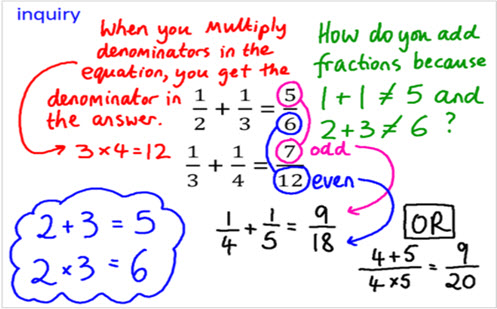Go to website

Throughout this inquiry students will come to identify and create patterns, conjecture and generalisations. They will learn how to add and subtract fractions with unrelated denominators and present fractions on a number line. Students are to identify multiples and factors of numbers to successfully add and subtract fractions. Teachers can use the inquiry as a teaching tool to model the worked examples and to probe questions. Students can record their own fraction inquiries to share, providing the teacher with an insight into the student's level of understanding. Supporting resources provided.

Year level(s) Year 7
Audience Student, Teacher
Purpose Assessment advice, Content knowledge, Evidence-based approaches, Teaching resource, Teaching strategies
Teaching strategies and pedagogical approaches Collaborative learning, Concrete Representational Abstract model, Differentiated teaching, Explicit teaching, Feedback, Growth mindset, Mathematics investigation, Metacognitive strategies, Questioning, Worked examples
Keywords fraction, numerator, denominator, equivalent fraction, common multiple, number line

## Curriculum alignment

Curriculum connections Critical and creative thinking, Numeracy
Strand and focus Algebra, Number, Apply understanding, Build understanding
Topics Addition and subtraction, Fractions, Multiples, factors and powers, Operating with number
AC: Mathematics (V9.0) content descriptions
AC9M7N06
Use the 4 operations with positive rational numbers including fractions, decimals and percentages to solve problems using efficient calculation strategies

Multiplicative strategies (P10)
Proportional thinking (P2)
Number and place value (P10)
Interpreting fractions (P8)